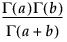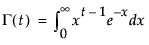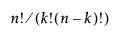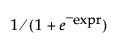JSL Syntax Reference > JSL Functions > Transcendental Functions
Publication date: 03/23/2021

# Transcendental Functions

Arrhenius(n)

Description

Converts the temperature n to the value of explanatory variable in Arrhenius model.

Returns

11604.5181215503/(n+273.15)

Argument

n

Temperature in Celsius.

Notes

This is frequently used as a transformation.

Arrhenius Inv(n)

Description

The inverse of the Arrhenius function. Converts the value n to the temperature in Celsius.

Returns

(11604.5181215503/n)-273.15

Argument

n

The value of the converted explanatory variable in Arrhenius model.

Notes

This is frequently used as a transformation.

Beta(a, b)

DescriptionReturns

Returns the beta function.

Arguments

a, b

numbers

Cytometry Logicle(x, T, W, M, A)

Description

Computes a cytometry logicle transformation.

See Also

Update for the logicle data scale including operational code implementations (Moore & Parks, 2012)

Cytometry Logicle Inverse(y, T, W, M, A)

Description

Computes the inverse cytometry logicle transformation.

See Also

Update for the logicle data scale including operational code implementations (Moore & Parks, 2012)

Digamma(n)

Description

The derivative of the log of the gamma function (LGamma).

Returns

The digamma function evaluated at n.

Argument

n

A number

Exp(a)

Description

Raises e to the power a.

Returns

ea.

Argument

a

A number

Equivalent Expression

`e()^a`

ExpM1(x)

Description

Returns a more accurate calculation of Exp(x)-1 when x is very small.

Factorial(n)

Description

Multiplies all numbers 1 through n, inclusive

Returns

The product.

Arguments

n

Any integer

Notes

One and only one argument must be specified.

FFT({list }, <named arguments>)

Description

Conducts a Fast Fourier Transformation (FFT) on a list of matrices.

Returns

The function takes one matrix, or a list of matrices for complex numbers. The returned value is a list of two matrices with the same dimensions as the first argument.

Argument

List

A list of one or two matrices. If one is provided, it is considered to be the real part. If two are provided, the first is the real part and the second is the imaginary part. Both matrices must have the same dimensions, and both must have more than one row.

Optional Named Arguments

<<inverse(Boolean)

If true (1), an inverse FFT is conducted.

<<multivariate(Boolean)

If true (1), a multivariate FFT is conducted. If false(0), a spatial FFT is conducted.

<<scale(number)

Multiplies the return values by the specified number.

Fit Transform To Normal(Distribution("name"), Y(vector), <Freq(vector))

Description

Fits a transformation to normality for a vector of data. This includes the Johnson Sl, Johnson Sb, Johnson Su, and GLog distributions.

Returns

A list that contains parameter estimates, the covariance matrix, the log-likelihood, AICc, a convergence message, and the transformed values. See Likelihood, AICc, and BIC in Fitting Linear Models.

Gamma(t, <limit>)

Description

The gamma function of x, or for each row if x is a column:Returns

The gamma.

Arguments

t

a number or a column

limit

optional limit. The default is infinity.

Notes

Gamma(t, limit) is the same integral as Gamma(t) but with the limit of integration that is defined instead of infinity.

LGamma(t)

Description

Returns the log gamma function for t, which is the natural log of gamma.

Ln(n)

Description

Returns the natural logarithm (base e logarithm) of n.

Log(n, <base>)

Description

Returns the natural logarithm (base e logarithm) of n. An optional second argument lets you specify a different base. For example, Log(n,3) for the base 3 logarithm of n. The Log argument can be any numeric expression. The expression Log(e()) evaluates as 1, and Log(32,2) is 5.

Log10(n)

Description

Returns the common (base 10) logarithm of n.

Log1P(n)

Description

Same as Log(1 + x), except that it is more accurate when x is very small.

Logist(x)

Description

Returns 1/(1+Exp(-x)), which converts a number in the domain -∞...+∞ into range 0...1. The function is useful in logistic regression.

Logist Percent(p)

Description

Similar to the Logist() function but with the result scaled from 0 to 100.

Logit(p)

Description

Returns log(p/(1-p)).

Logit Percent(p)

Description

Similar to the Logit() function with the argument 0 to 100 rather than 0 to 1.

N Choose K(n, k)

Description

This function returns the number of n things taken k at a time (“n choose k”) and is computed in the standard way using factorials, as. For example, NChooseK(5,2) evaluates as 10.

Notes

This is implemented internally in JMP using lGamma functions. The result is not always an integer.

Power(a, <b>)

a^b

Description

Raises a to the power of b.

Returns

The product of a multiplied by itself b times.

Arguments

a

Can be a variable, number, or matrix.

b

(Optional) Can be a variable or a number.

Notes

For Power(), the second argument (b) is optional, and the default value is 2. Power(a) returns a2.

Root(n, <r>)

Description

Returns the rth root of n, where r defaults to 2 for square root.

SbInv(z, gamma, delta, theta, sigma)

Description

Returns a transformation of a standard normal variable to a double bounded Johnson variable.

SbTrans(x, gamma, delta, theta, sigma)

Description

Returns a transformation of a double bounded Johnson variable to a standard normal variable.

Scheffe Cubic(x1, x2)

Description

Returns x1*x2*(x1-x2). This function supports notation for cubic mixture models.

SHASHInv(z, gamma, delta, theta, sigma)

Description

Returns a transformation of a standard normal variable to a sinh-arcsinh (SHASH) variable. The transformation is calculated as σ*sinh((arcsinh(z)-γ)/δ)+θ.

SHASHTrans(x, gamma, delta, theta, sigma)

Description

Returns a transformation of a sinh-arcsinh (SHASH) variable to a standard normal variable. The transformation is calculated as sinh(γ+δ*arcsinh((x-θ)/σ)).

SlInv(z, gamma, delta, theta, sigma)

Description

Returns a transformation of a standard normal variable to a Johnson Sl variable.

SlTrans(x, gamma, delta, theta, sigma)

Description

Returns a transformation of a Johnson Sl variable to a standard normal variable.

Sqrt(n)

Description

Returns the square root of n.

Squash(expr)

Description

An efficient computation of the function 1/ [1 + exp(expr)].

Squish(expr)

Description

Equivalent to Squash(-expr), or.

SuInv(z, gamma, delta, theta, sigma)

Description

Returns a transformation of a standard normal variable to an unbounded Johnson variable.

SuTrans(x, gamma, delta, theta, sigma)

Description

Returns a transformation of an unbounded Johnson variable to a standard normal variable.

Trigamma()

Description

Returns the trigamma function evaluated at n. The trigamma function is the derivative of the digamma function.

Want more information? Have questions? Get answers in the JMP User Community (community.jmp.com).